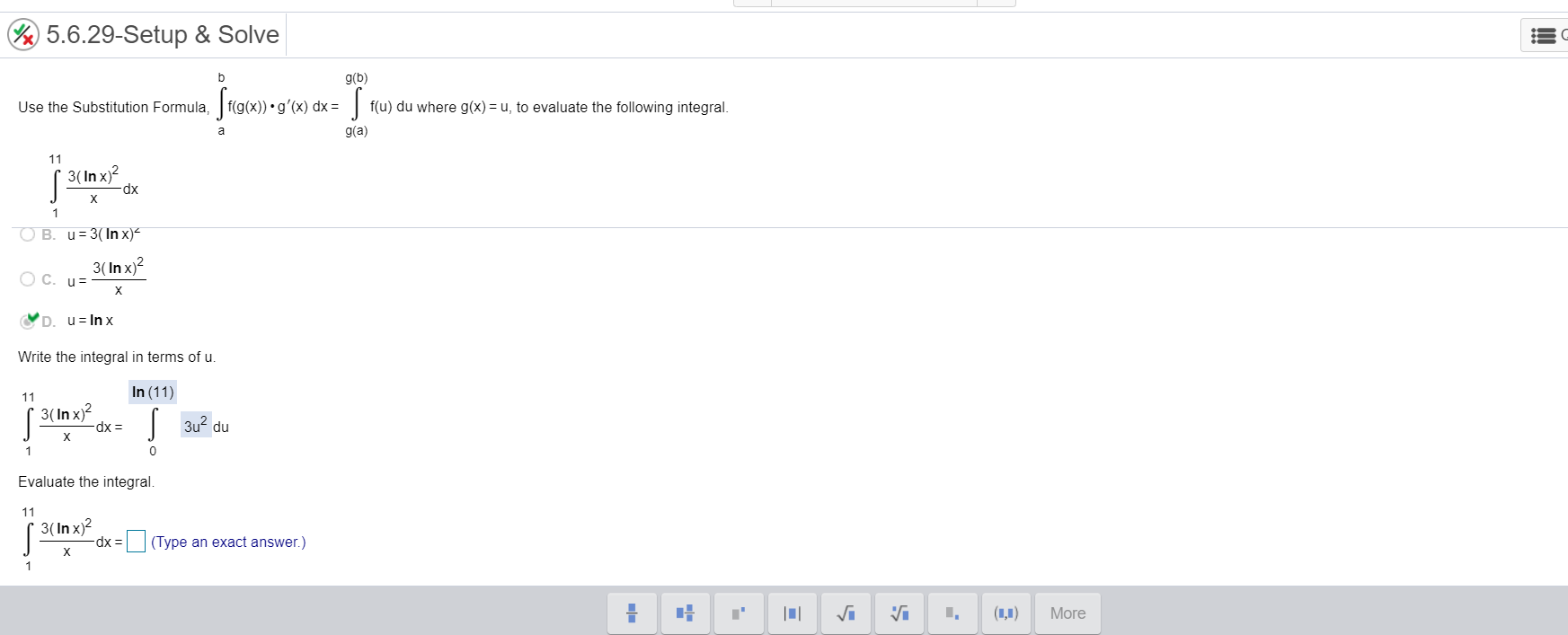# 5.6.29-Setup & Solveg(b)f(u) du where g(x) = u, to evaluate the following integral.f(g(x)) •g'(x) dx =Use the Substitution Formula,g(a)a113( In x)?dp-1O B. u= 3(In x)-3( In x)?O C. u=X.D. u= In xWrite the integral in terms of u.In (11)113( In x)?-dx =| 3u? duEvaluate the integral.113( In x)?dx =(Type an exact answer.)(1,1)More

Question
1 views

I need help evaluating the integral.help_outlineImage Transcriptionclose5.6.29-Setup & Solve g(b) f(u) du where g(x) = u, to evaluate the following integral. f(g(x)) •g'(x) dx = Use the Substitution Formula, g(a) a 11 3( In x)? dp- 1 O B. u= 3(In x)- 3( In x)? O C. u= X. D. u= In x Write the integral in terms of u. In (11) 11 3( In x)? -dx = | 3u? du Evaluate the integral. 11 3( In x)? dx =(Type an exact answer.) (1,1) More fullscreen
check_circle

Step 1

We need to evaluate the given definite i...

### Want to see the full answer?

See Solution

#### Want to see this answer and more?

Solutions are written by subject experts who are available 24/7. Questions are typically answered within 1 hour.*

See Solution
*Response times may vary by subject and question.
Tagged in

### Integration